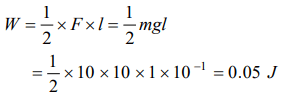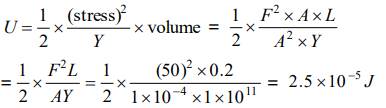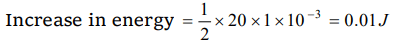## Mechanical Properties of Solids Questions and Answers Part-17

1. A 5 metre long wire is fixed to the ceiling. A weight of 10 kg is hung at the lower end and is 1 metre above the floor. The wire was elongated by 1 mm. The energy stored in the wire due to stretching is
a) Zero
b) 0.05 joule
c) 100 joule
d) 500 joule

Explanation:2. If the force constant of a wire is K, the work done in increasing the length of the wire by l is
a) Kl/2
b) Kl
c) $Kl^{2}\diagup 2$
d) $Kl^{2}$

Explanation:3. If the tension on a wire is removed at once, then
a) It will break
b) Its temperature will reduce
c) There will be no change in its temperature
d) Its temperature increases

Explanation: Due to tension, intermolecular distance between atoms is increased and therefore potential energy of the wire is increased and with the removal of force interatomic distance is reduced and so is the potential energy. This change in potential energy appears as heat in the wire and thereby increases the temperature.

4. When strain is produced in a body within elastic limit, its internal energy
a) Remains constant
b) Decreases
c) Increases
d) None of the above

Explanation: Due to increase in intermolecular distance

5. When shearing force is applied on a body, then the elastic potential energy is stored in it. On removing the force, this energy
a) Converts into kinetic energy
b) Converts into heat energy
c) Remains as potential energy
d) None of the above

Explanation: Converts into heat energy

6. A brass rod of cross-sectional area 1 cm2 and length 0.2 m is compressed lengthwise by a weight of 5 kg. If Young's modulus of elasticity of brass is $1\times 10^{11}N\diagup m^{2}$   and $g= 10m\diagup sec^{2}$ , then increase in the energy of the rod will be
a) $10^{-5}J$
b) $2.5\times 10^{-5}J$
c) $5\times 10^{-5}J$
d) $2.5\times 10^{-4}J$

Explanation:7. If one end of a wire is fixed with a rigid support and the other end is stretched by a force of 10 N,then the increase in length is 0.5 mm. The ratio of the energy of the wire and the work done in displacing it through 1.5 mm by the weight is
a) $\frac{1}{3}$
b) $\frac{1}{4}$
c) $\frac{1}{2}$
d) 1

Explanation:8. A wire is suspended by one end. At the other end a weight equivalent to 20 N force is applied. If the increase in length is 1.0 mm, the increase in energy of the wire will be
a) 0.01 J
b) 0.02 J
c) 0.04 J
d) 1.00 J

Explanation:9. In the above question, the ratio of the increase in energy of the wire to the decrease in gravitational potential energy when load moves downwards by 1 mm, will be
a) 1
b) $\frac{1}{4}$
c) $\frac{1}{3}$
d) $\frac{1}{2}$b) $\sqrt{2EY}$
c) $\sqrt{\frac{2E}{Y}}$
d) $\frac{E}{Y}$